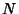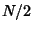## Danielson-Lanczos Lemma

The Discrete Fourier Transform of length(whereis Even) can be rewritten as the sum of two Discrete Fourier Transforms, each of length. One is formed from the Even-numbered points; the other from the Odd-numbered points. Denote theth point of the Discrete Fourier Transform by. Thenwhereand. This procedure can be applied recursively to break up theeven and Odd points to theirEven and Odd points. Ifis a Power of 2, this procedure breaks up the original transform intotransforms of length 1. Each transform of an individual point hasfor some. By reversing the patterns of evens and odds, then lettingand, the value ofin Binary is produced. This is the basis for the Fast Fourier Transform.